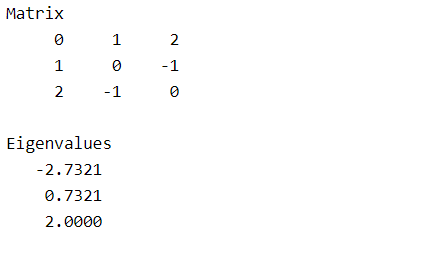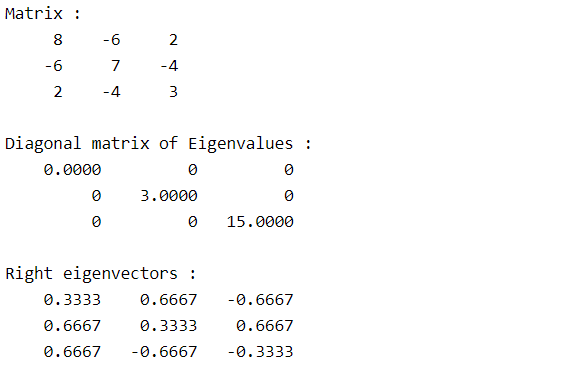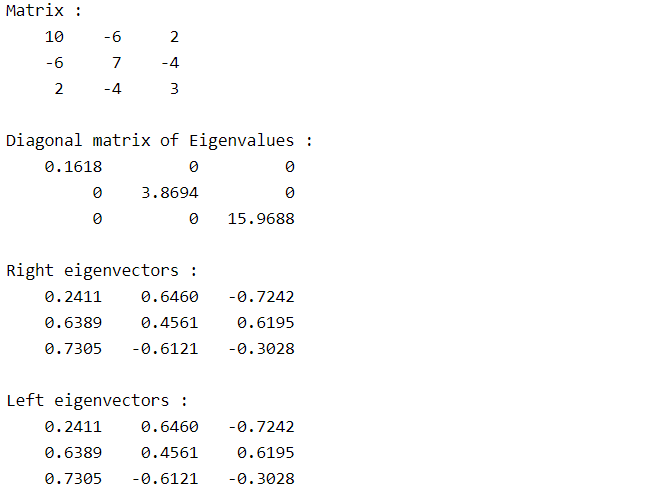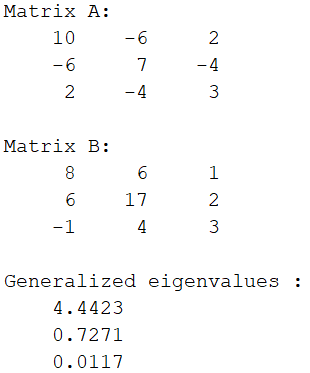Related Articles

# Eigenvalues and Eigenvectors in MATLAB

• Last Updated : 14 May, 2021

Eigenvalues and Eigenvectors are properties of a square matrix.

Letis an N*N matrix, X be a vector of size N*1 andbe a scalar.

Then the values X,satisfying the equationare eigenvectors and eigenvalues of matrix A respectively.

• A matrix of size N*N possess N eigenvalues
• Every eigenvalue corresponds to an eigenvector.

Matlab allows the users to find eigenvalues and eigenvectors of matrix using eig() method. Different syntaxes of eig() method are:

• e = eig(A)
• [V,D] = eig(A)
• [V,D,W] = eig(A)
• e = eig(A,B)

Let us discuss the above syntaxes in detail:

### e = eig(A)

• It returns the vector of eigenvalues of square matrix A.

## Matlab

 % Square matrix of size 3*3A = [0 1 2;    1 0 -1;    2 -1 0];disp("Matrix");disp(A);  % Eigenvalues of matrix Ae = eig(A);disp("Eigenvalues");disp(e);

Output :### [V,D] = eig(A)

• It returns the diagonal matrix D having diagonals as eigenvalues.
• It also returns the matrix of right vectors as V.
• Normal eigenvectors are termed as right eigenvectors.
• V is a collection of N eigenvectors of each N*1 size(A is N*N size) that satisfies A*V = V*D

## Matlab

 % Square matrix of size 3*3A = [8 -6 2;    -6 7 -4;    2 -4 3];disp("Matrix");disp(A);  % Eigenvalues and right eigenvectors of matrix A[V,D] = eig(A);disp("Diagonal matrix of Eigenvalues");disp(D);disp("Right eigenvectos")disp(V);

Output :### [V,D,W] = eig(A)

• Along with the diagonal matrix of eigenvalues D and right eigenvectors V, it also returns the left eigenvectors of matrix A.
• A left eigenvector u is a 1*N matrix that satisfies the equation u*A = k*u, where k is a left eigenvalue of matrix A.
• W is the collection of N left eigenvectors of A that satisfies W’*A = D*W’.

## Matlab

 % Square matrix of size 3*3A = [10 -6 2;    -6 7 -4;     2 -4 3];disp("Matrix :");disp(A);  % Eigenvalues and right and left eigenvectors % of matrix A[V,D,W] = eig(A);disp("Diagonal matrix of Eigenvalues :");disp(D);disp("Right eigenvectors :")disp(V);disp("Left eigenvectors :")disp(W);

Output :### e = eig(A,B)

• It returns the generalized eigenvalues of two square matrices A and B of the same size.
• A generalized eigenvalue λ and a corresponding eigenvector v satisfy Av=λBv.

## Matlab

 % Square matrix A and B of size 3*3A = [10 -6 2;    -6 7 -4;     2 -4 3];B = [8 6 1;     6 17 2;    -1 4 3];      disp("Matrix A:");disp(A);disp("Matrix B:");disp(B);  % Generalized eigen values % of matrices A and Be = eig(A,B);disp("Generalized eigenvalues :")disp(e);

Output :Attention reader! Don’t stop learning now. Get hold of all the important DSA concepts with the DSA Self Paced Course at a student-friendly price and become industry ready.  To complete your preparation from learning a language to DS Algo and many more,  please refer Complete Interview Preparation Course.

In case you wish to attend live classes with experts, please refer DSA Live Classes for Working Professionals and Competitive Programming Live for Students.

My Personal Notes arrow_drop_up